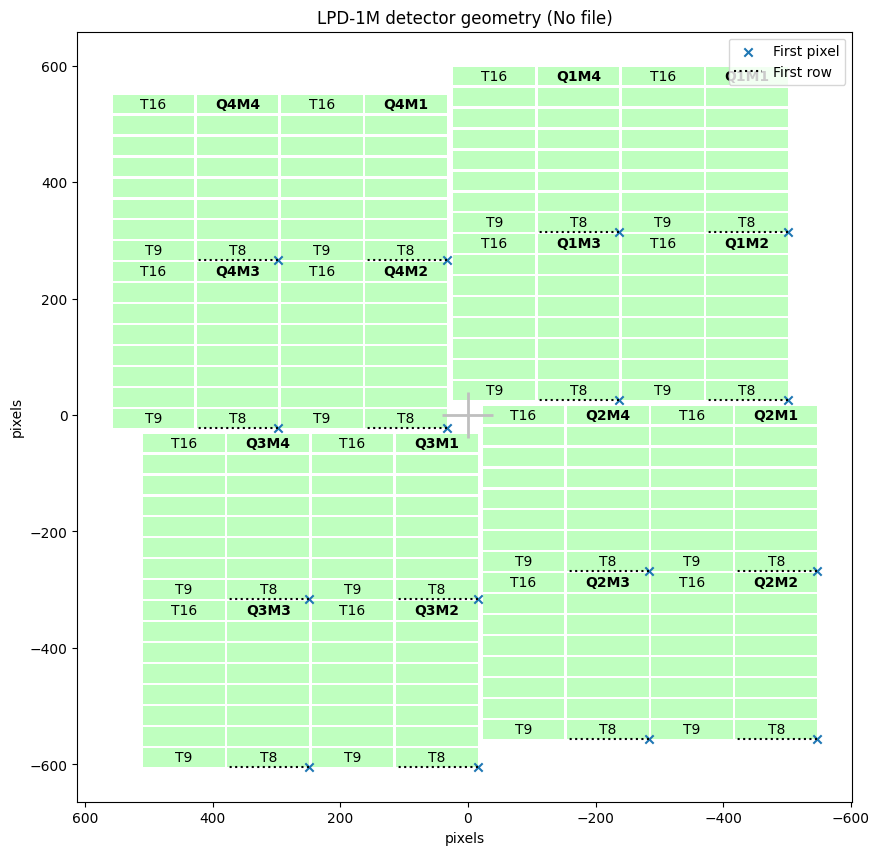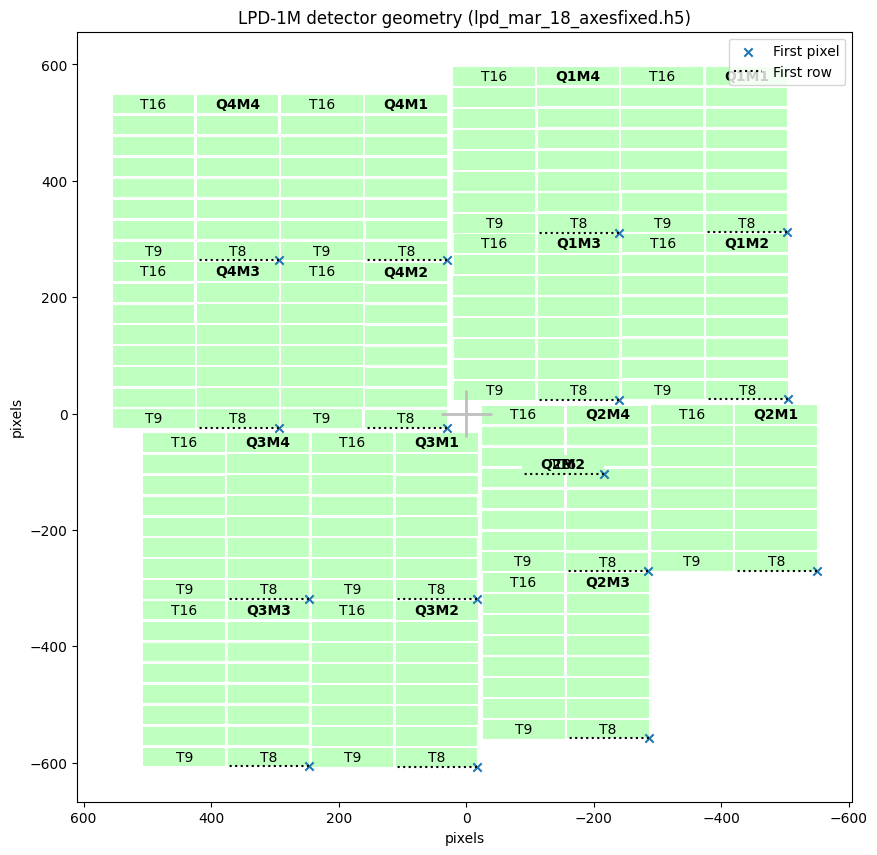# LPD-1M geometry¶

The LPD-1M detector, used in FXE, consists of 16 supermodules of 256×256 pixels each. Each module is divided into 16 tiles.

:

%matplotlib inline
from extra_geom import LPD_1MGeometry


We can generate a simple geometry from (x, y) coordinates of the top, beam-left (left looking along the beam) corner of each quadrant:

:

quadpos = [(11.4, 299), (-11.5, 8), (254.5, -16), (278.5, 275)]  # in mm
geom.inspect()

:

<matplotlib.axes._subplots.AxesSubplot at 0x2afb34c5af28>We can also load geometry information within each quadrant from an EuXFEL HDF5 geometry file. These files do not store the quadrant positions, so we need to use it along with the quadrant positions, in the same format as above.

:

# From March 18; converted to XFEL standard coordinate directions

:

<matplotlib.axes._subplots.AxesSubplot at 0x2afb34b9dc18>